Search datasheet (1.687.043 components) Search fieldPart namePart descriptionAbout site Manufacturers list ChipFindIC search engine AllXrefCross-reference database### Datasheet: F0321818M (Eudyna Devices Inc.)

2 Ghz Bandwidth Limiting Amplifier
Download:PDFZIP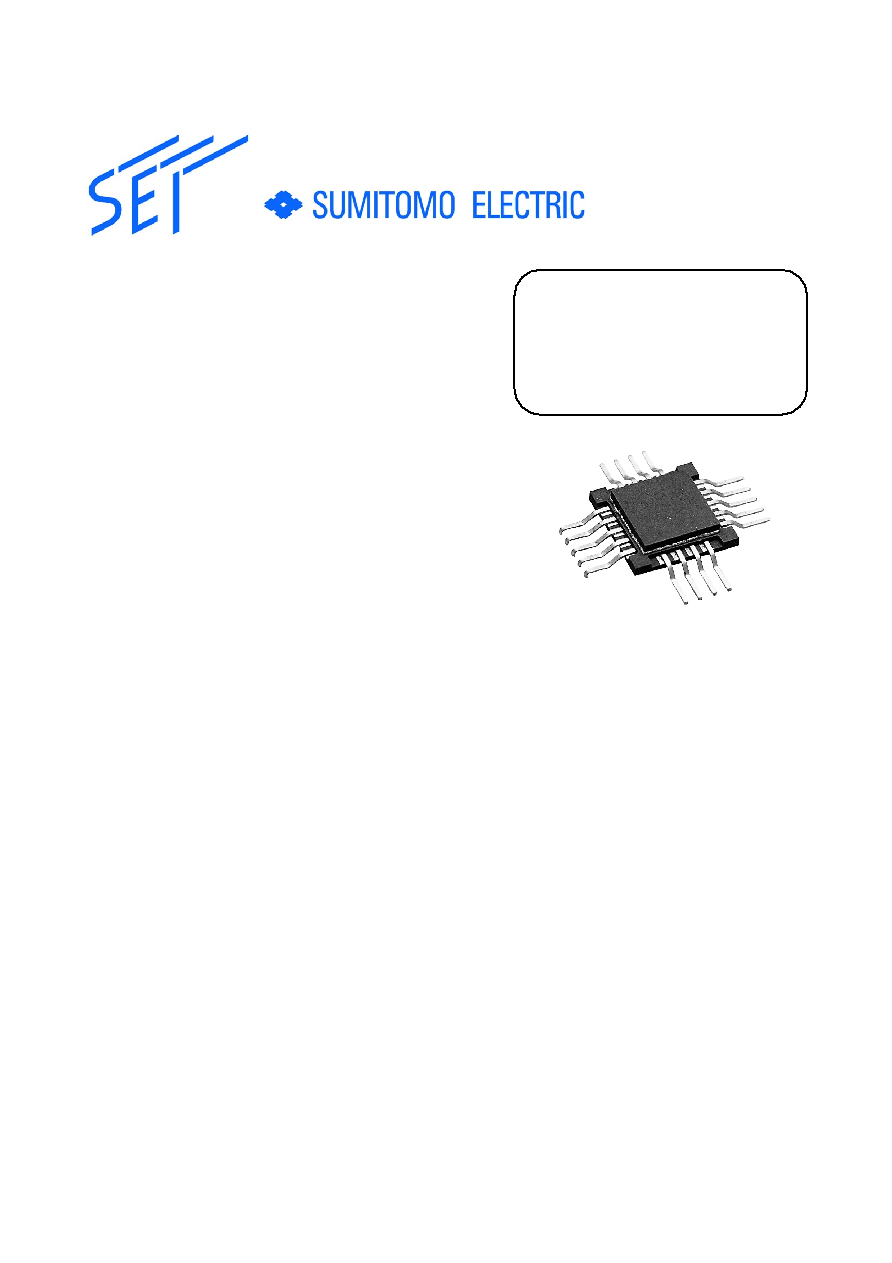Features
� +5 V single power supply
� 20 dB typical gain
� 2.0 GHz typical -3 dB cutoff frequency
� On-chip matching to 50
� 55 mA typical operating current
� Differential input and output
� Differential ECL compatible input
Applications
� Post-amplifier of an optical receiver circuit
up to 2.5 Gb/s
� Logic gate buffer to interface between analog circuit and logic circuit
Functional Description
The F0321818M is a stable GaAs integrated limiting amplifier for use in a post-amplifier
of an optical receiver circuit up to 2.5 Gb/s. The F0321818M typically specifies a small signal
gain of 20 dB (Rs=RL=50
) with a 3 dB-cutoff-frequency of 2.0 GHz. It features single +5 V
supply operation, excellent VSWR's of 1.1:1, and a typical dissipation current of 55 mA.
The F0321818M can be also used as interface circuits in sensing systems and measure-
ment instruments. Emitter coupled logic (ECL) or source coupled FET logic (SCFL) circuits
are the most popular IC's for high speed digital circuits; the F0321818M operating under a
differential ECL compatible input condition is the best choice as the interface IC to join ana-
log circuits to ECL circuits or conventional GaAs logic IC's.
Limiting Amplifier
F0321818M
2 GHz Bandwidth
01.08.28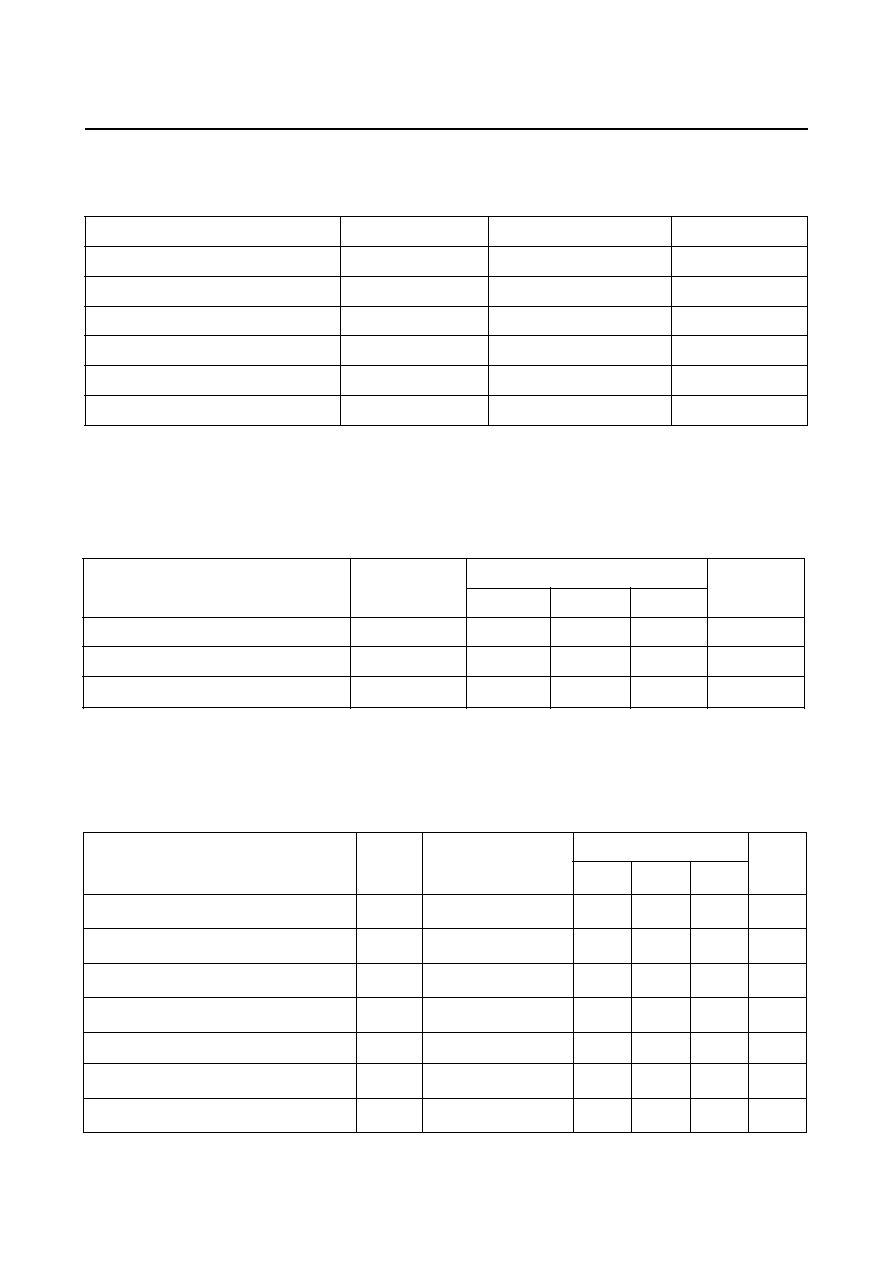F0321818M
2 GHz Limiting Amplifier
Absolute Maximum Ratings
T
a
=25
C, unless specified
Recommended Operating Conditions
V
SS
= GND
Electrical Characteristics
T
a
= 25
C, V
DD
= +5V, V
SS
= GND, unless specified
r
e
t
e
m
a
r
a
P
l
o
b
m
y
S
e
u
l
a
V
s
ti
n
U
.
n
i
M
.
p
y
T
.
x
a
M
e
g
a
tl
o
V
y
l
p
p
u
S
V
D
D
5
7
.
4
5
6
4
.
5
V
d
a
o
L
d
e
l
p
u
o
C
C
A
L
R
-
0
5
-
e
r
u
t
a
r
e
p
m
e
T
g
n
it
a
r
e
p
O
t
n
e
i
b
m
A
T
a
0
5
2
0
7
C
Parameter
Symbol
Test Conditions
Value
Units
Min.
Typ.
Max.
Supply Current
Input Bias Point
Output bias Point
VSWR (IN, OUT)
Gain
-3dB High Frequency Cutoff
Maximum Output Swing (single output)
I
DD
V
IN
V
OUT
SWR
GV
Fc
Vom
Pin=-40dBm
Pin=-40dBm f=1MHz
Pin=-40dBm
RL=50
f=1MHz
Pin=-40dBm
RL=50
RL=50
-
-
-
-
18
1.8
0.4
55
1.5
3.5
1.1
20
2.0
0.6
70
-
-
1.8
-
-
0.8
mA
V
V
-
dB
GHz
V
r
e
t
e
m
a
r
a
P
l
o
b
m
y
S
e
u
l
a
V
s
t
i
n
U
e
g
a
t
l
o
V
y
l
p
p
u
S
V
D
D
V
S
S
V
o
t
5
.
0
-
S
S
7
+
V
t
n
e
r
r
u
C
y
l
p
p
u
S
I
D
D
0
8
A
m
)
C
A
(
g
n
i
w
S
e
g
a
t
l
o
V
t
u
p
n
I
V
,
+
N
I
V
-
N
I
1
V
e
g
a
t
l
o
V
t
u
p
t
u
O
V
,
+
T
U
O
V
-
T
U
O
V
D
D
V
o
t
5
.
2
-
D
D
V
e
r
u
t
a
r
e
p
m
e
T
g
n
i
t
a
r
e
p
O
t
n
e
i
b
m
A
T
a
0
7
+
o
t
0
C
e
r
u
t
a
r
e
p
m
e
T
e
g
a
r
o
t
S
T
g
t
s
5
2
1
+
o
t
5
5
-
C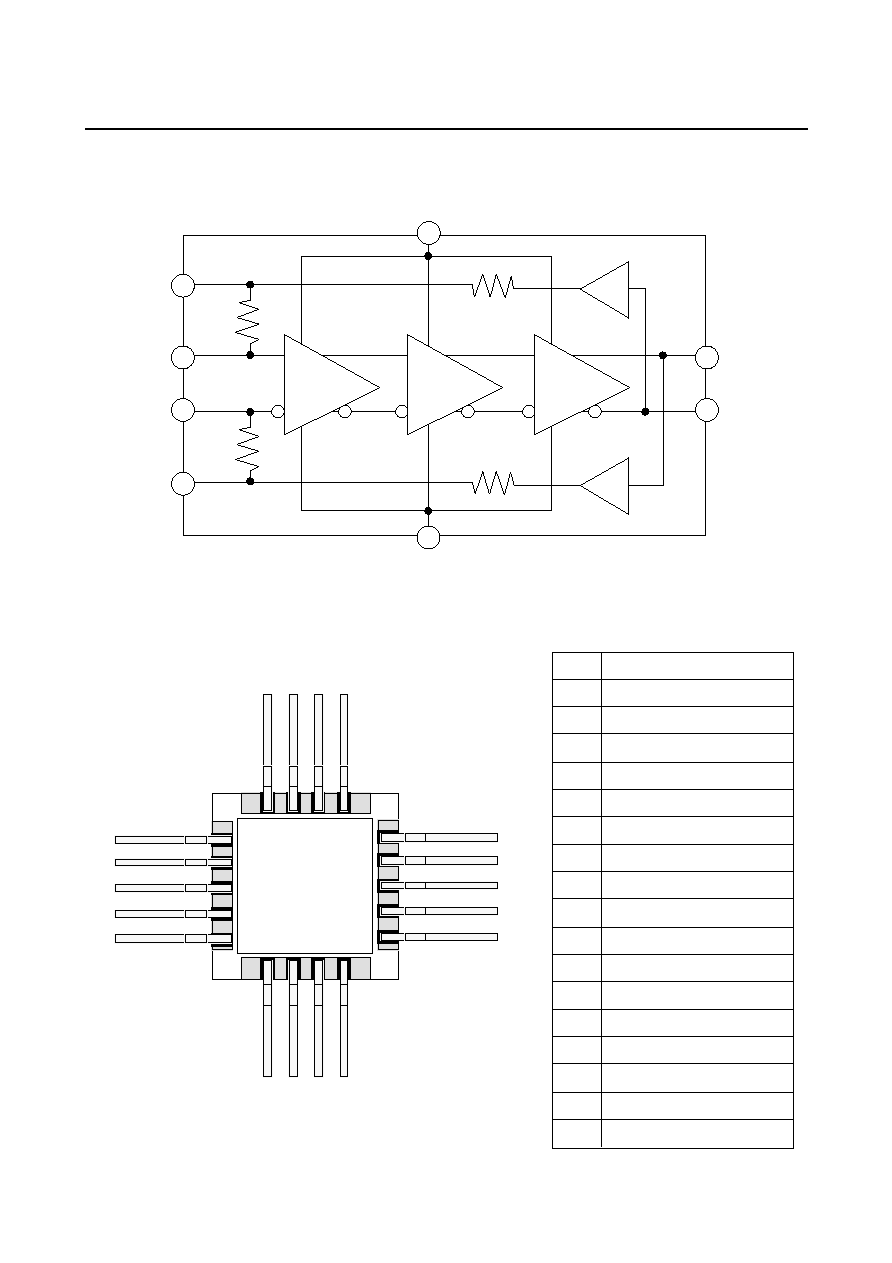2 GHz Limiting Amplifier
F0321818M
Block Diagram
Pin Assignments (Top View)
Pin Descriptions
V
DD
V
SS
V
OUT-
V
OUT+
V
IN+
V
IN-
LPF-
LPF+
Amp.
Amp.
Output
Buffer
1
2
3
4
5
6
7
8
9
10
11
12
13
14
15
16
17
18
NOTE: Package bottom is Internally connected to V
SS
1
VSS Supply Voltage
2
VOUT- Output
3
VSS Supply Voltage
4
5
6
7
LPF - AC Ground
8
VIN- Input
9
10
VIN+ Input
11
LPF+ AC Ground
12
VSS Supply Voltage
13
VDD Supply Voltage
14
VDD Supply Voltage
15
16
VOUT+ Output
17
18
VSS Supply Voltage
VSS Supply Voltage
VSS Supply Voltage
VSS Supply Voltage
VSS Supply Voltage
VSS Supply Voltage
VSS Supply Voltage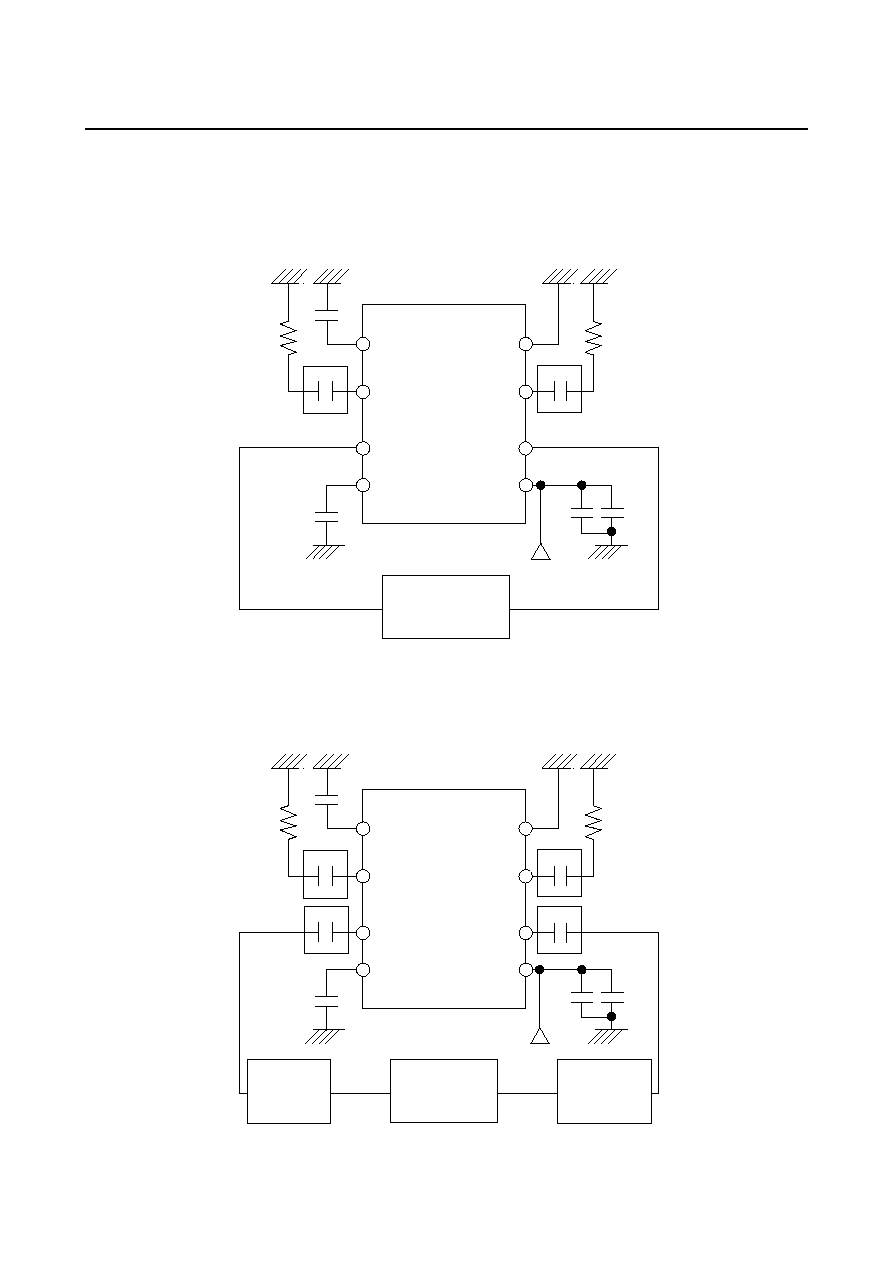F0321818M
2 GHz Limiting Amplifier
Test Circuits
*
*
50
50
1) AC Characteristics
F0321818M
LPF-
V
SS
V
IN-
V
OUT-
LPF+
V
DD
V
DD
V
IN+
V
OUT+
0.1
F
0.1
F
0.1
F
Pin =-40 dBm
2200
pF
Vector
Network
Analyzer
50
50
2) Limiting Characteristics
*
*
*
*
50
50
50
LPF-
V
SS
V
IN-
V
OUT-
LPF+
V
DD
V
DD
V
IN+
V
OUT+
0.1
F
0.1
F
0.1
F
2200
pF
Pulse
Pattern
Generator
Attenuator
Sampling
Oscilloscope
DATA
CLOCK
* DC BLOCK
(PICOSECIND PULSE LABS, MODEL 5501)
F0321818M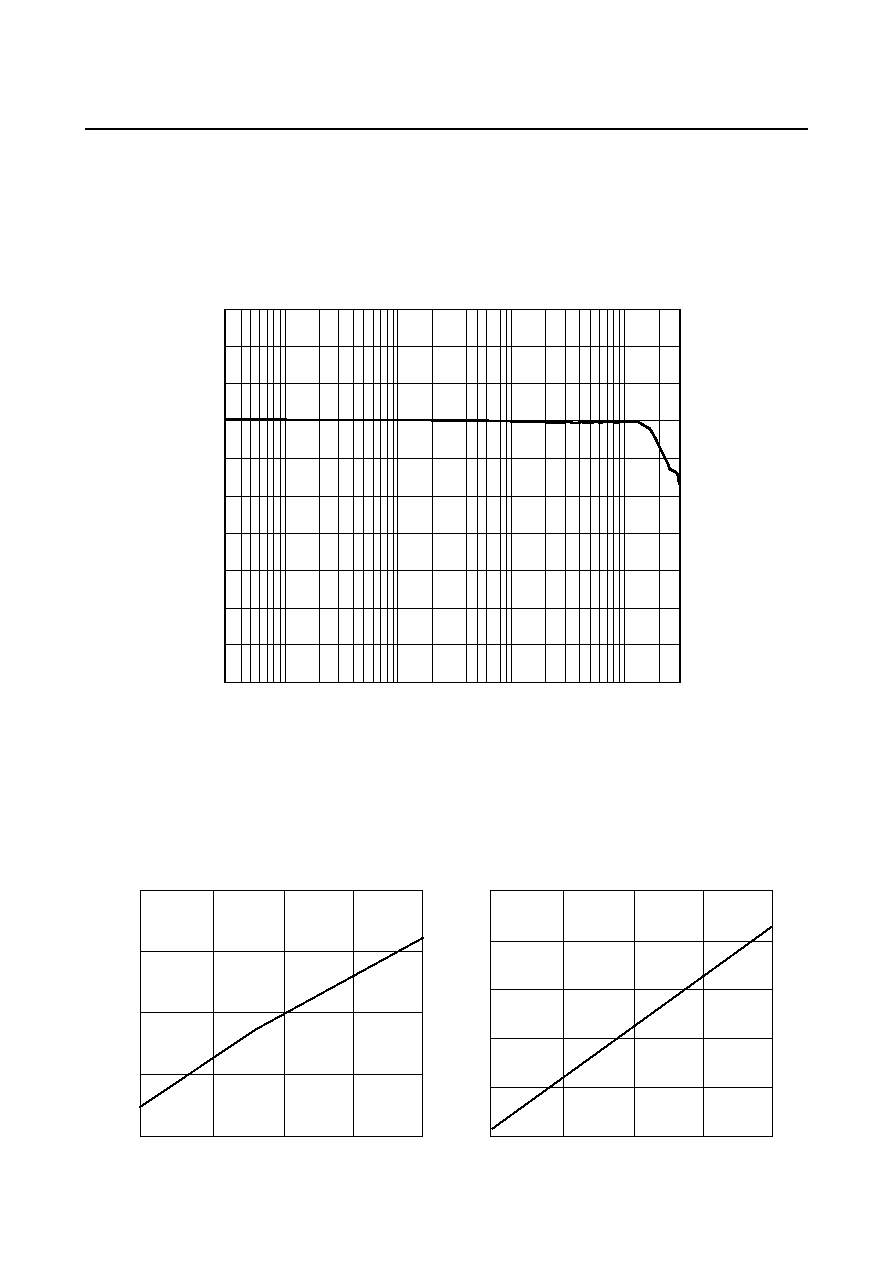2 GHz Limiting Amplifier
F0321818M
Typical AC Characteristics
(1) Gain
T
a
=25
C, V
DD
=+5 V, V
SS
=GND, Pin =-40 dBm, RL =50
, 300 kHz-3 GHz
(2) Dependance of Gain and High Frequency Cutoff on Power Supply Variations
T
a
=25
C, V
DD
=-5 V, V
SS
=GND, Pin =-40 dBm, RL =50
, 300 kHz-3 GHz
19
20
21
22
23
Gain (dB)
High Frequency Cut-off (GHz)
4.5
5.0
5.5
V
DD
(V)
4.5
5.0
5.5
V
DD
(V)
2.10
2.12
2.14
2.16
2.18
2.20
1M
10M
100M
1G
Frequency (Hz)
0
9
18
27
(dB)© 2020 • ICSheet• Contact form• Main page